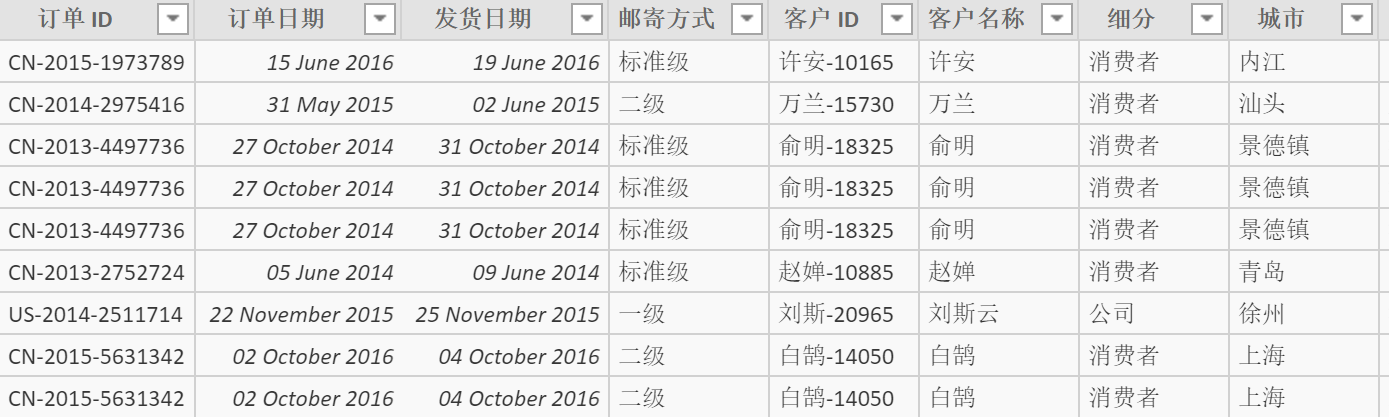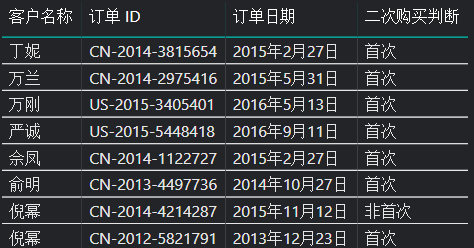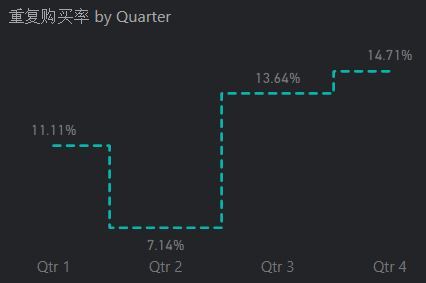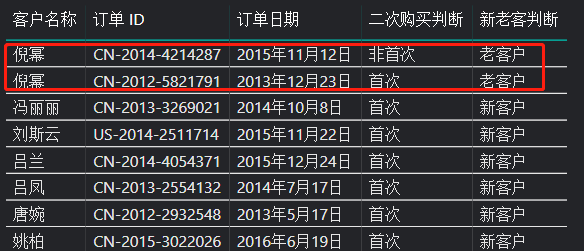# DAX计算重复购买率与新老客自动分类

## 目标

1.把订单分为客户首次购买的订单和后续购买的订单，进而算出重复购买率 2.把客户分为新客户与老客户，进而计算新老客占比

## 过程``````二次购买判断 =
VAR
E_Date = 'Data'[订单日期]
VAR
CUST = 'Data'[客户 ID]
RETURN
IF(
SUMX(
FILTER('Data',CUST = 'Data'[客户 ID]&&E_Date > 'Data'[订单日期]),
COUNTROWS('Data'))>0,"非首次","首次")
````````````重复购买率 =
DIVIDE(
CALCULATE(
DISTINCTCOUNT(Data[客户 ID]),'Data'[二次购买判断] = "非首次"),
DISTINCTCOUNT(Data[客户 ID])
)
``````• 把订单分为客户首次购买的订单和后续购买的订单，进而算出重复购买率
• 把客户分为新客户与老客户，进而计算新老客占比

``````新老客判断 =
if(
calculate(
distinctcount('Data'[订单日期]),
filter('Data','Data'[客户 ID]=earlier('Data'[客户 ID])))>1,
"老客户","新客户")
````````````老客户占比 =
DIVIDE(
CALCULATE(
DISTINCTCOUNT(Data[客户 ID]),'Data'[新老客判断] = "老客户"),
DISTINCTCOUNT(Data[客户 ID]))
``````
``````新客户占比 =
DIVIDE(
CALCULATE(
DISTINCTCOUNT(Data[客户 ID]),
'Data'[新老客判断] = "新客户"),
DISTINCTCOUNT(Data[客户 ID]))
``````
• 把订单分为客户首次购买的订单和后续购买的订单，进而算出重复购买率
• 把客户分为新客户与老客户，进而计算新老客占比

## 其他